# Suppose A is an n by n matrix that is diagonalized by P. Find a matrix that diagonalizes A^T

Suppose A is an n by n matrix that is diagonalized by P. Find a matrix that diagonalizes AT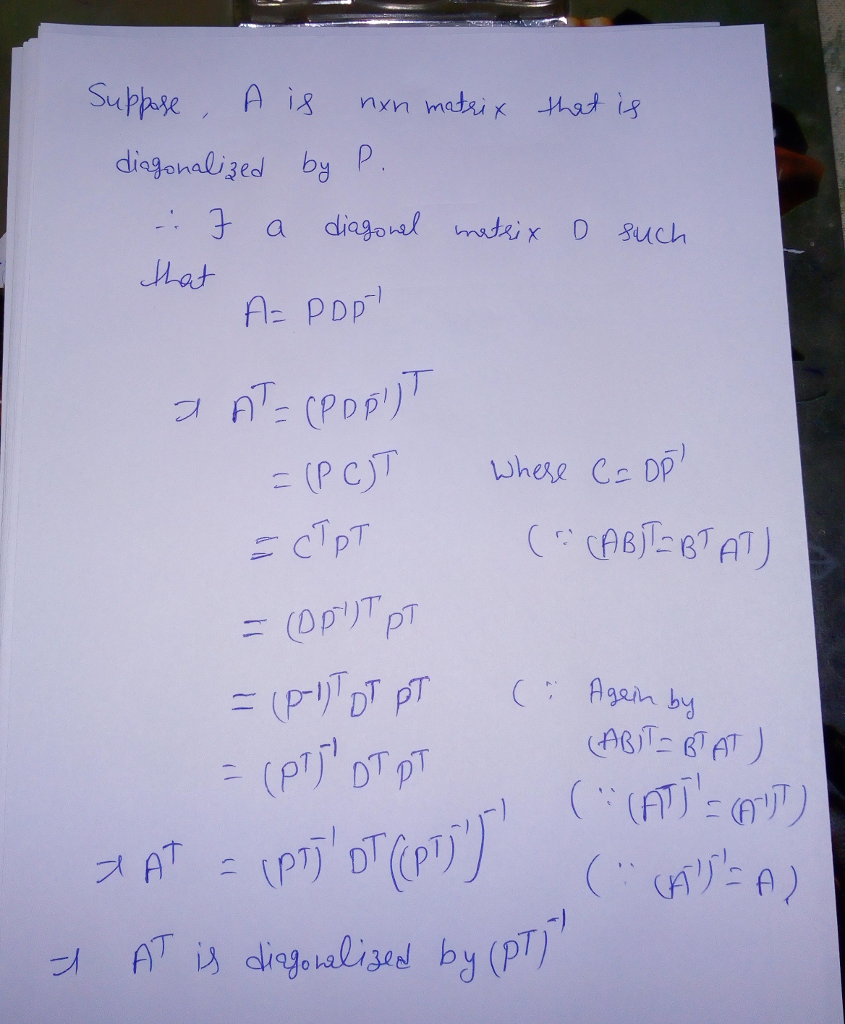#### Earn Coin

Coins can be redeemed for fabulous gifts.

Similar Homework Help Questions
• ### QUESTION) two matrix A,B can be simultaneously diagonalized if there is an invertible matrix that diagonalizes...

QUESTION) two matrix A,B can be simultaneously diagonalized if there is an invertible matrix that diagonalizes both of them 1) if A and B can be diagonalized, show that AB=BA 2)Conversely, if A and B, and if one of these matrices, say A, has distinct eigenvalues, show how they can be simultaneously diagonalized

• ### 9) Find a matrix P that diagonalizes A, and find P- AP 9) Find a matrix P that diagonalizes A, and find P- AP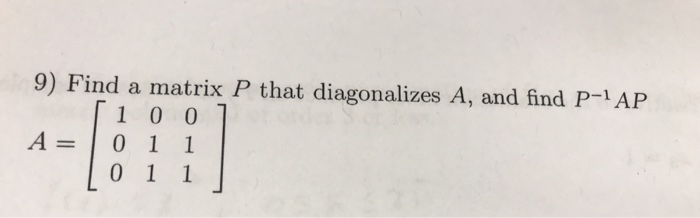9) Find a matrix P that diagonalizes A, and find P- AP 9) Find a matrix P that diagonalizes A, and find P- AP

• ### 1 2 Suppose that the non-singular n × n matrix A can be diagonalized, ie A...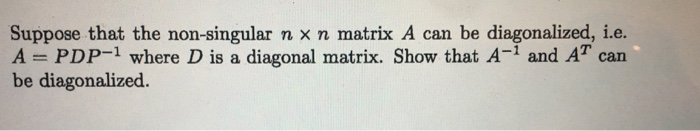1 2 Suppose that the non-singular n × n matrix A can be diagonalized, ie A = PDP-1 where D is a diagonal matrix. Show that A-1 and AT can be diagonalized. 1.e. Suppose we have 2nu x 2n block matrices Y=I-I B O AB where all sub-matrices are n × n and O denotes the zero matrix. Find a block matrix X such that XY the determinant of X? Z and demonstrate it works. What is

• ### I need help with two of these problems Find a matrix P that diagonalizes A =...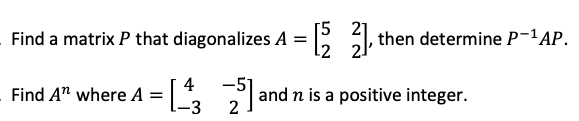I need help with two of these problems Find a matrix P that diagonalizes A = [ 2], then determine P-1AP. Find A” where A = [~ -5 2 and n is a positive integer. -3

• ### Find an orthogonal matrix P such that  diagonalizes . ​ a. ​ b. ​ c. ​ d....

Find an orthogonal matrix P such that  diagonalizes . ​ a. ​ b. ​ c. ​ d. ​ e. ​

• ### Linear Algebra - Find a matrix P that diagonalizes A, and compute P-1AP .

Find a matrix P that diagonalizes A, and compute P−1AP .[9 0 8][0 10 0][0 0 10]Enter the following as matrices, find the P with the smallest possible integer valuesAnd then it asks me to find the P-1and p-1APIve tried many times and keep getting it wrong, Thanks.

• ### Find an orthogonal matrix P such that PPAP diagonalizes A = - Lozi 13 2 V2...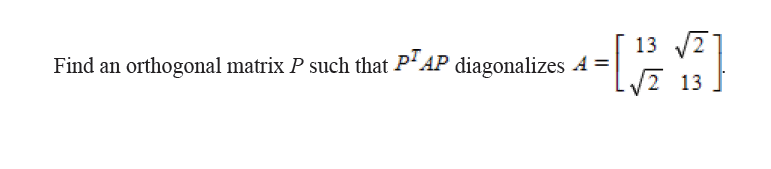Find an orthogonal matrix P such that PPAP diagonalizes A = - Lozi 13 2 V2 13

• ### 2. For the following matrices, find a matrix Pthat diagonalizes A and compute p-'AP. If the...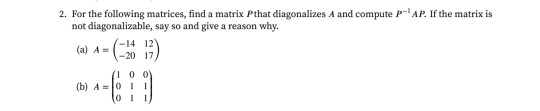2. For the following matrices, find a matrix Pthat diagonalizes A and compute p-'AP. If the matrix is not diagonalizable, say so and give a reason why. -14 12 (a) A= -2017 100 (b) A011 011

• ### I need help with two of these problems Find a matrix P that orthogonally diagonalizes A...I need help with two of these problems Find a matrix P that orthogonally diagonalizes A = -1 201 2 02, then find PT AP. 0 2 1] 3 a alw /10 Given that A is orthogonal, what is the value of a? 1 3 V10 V10

• ### Problem #9: Let 0 0 (a) Find a unitary matrix P that diagonalizes A (b) Find B - P-1AP using the ...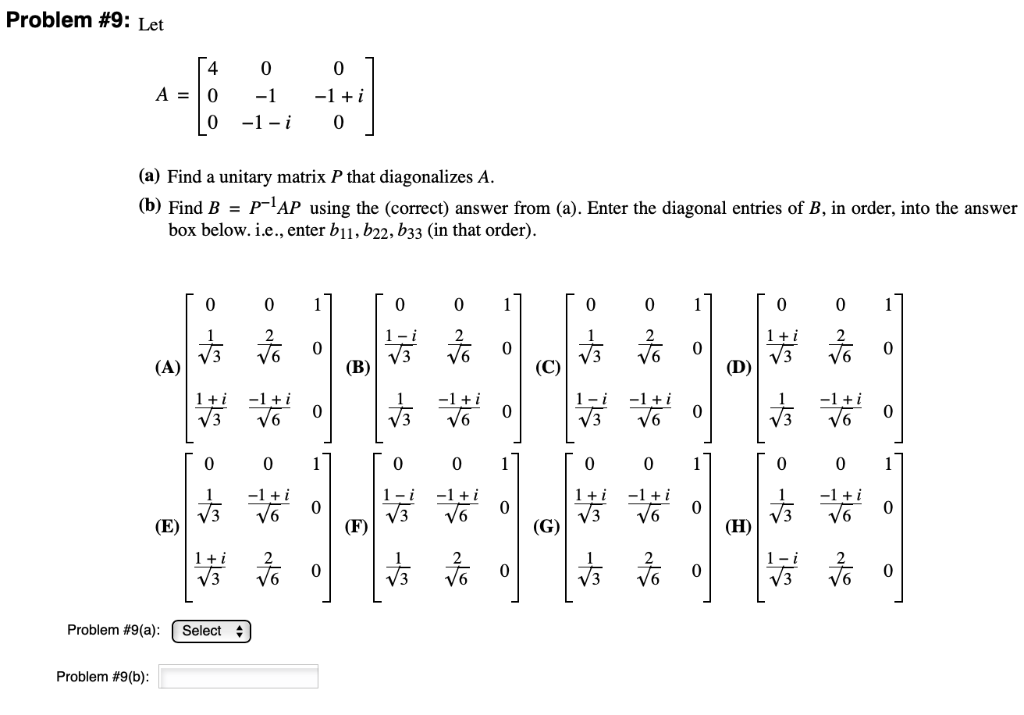Problem #9: Let 0 0 (a) Find a unitary matrix P that diagonalizes A (b) Find B - P-1AP using the (correct) answer from (a). Enter the diagonal entries of B, in order, into the answer box below. i.е., enter b11, b22, b33 (in that order). 0 0 0 0 0 0 0 0 из 6-0 Problem #9(a): | Select Problem #9(b) Problem #9: Let 0 0 (a) Find a unitary matrix P that diagonalizes A (b) Find B -...

Free Homework App